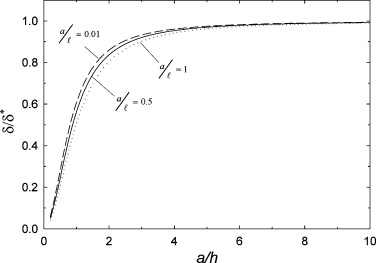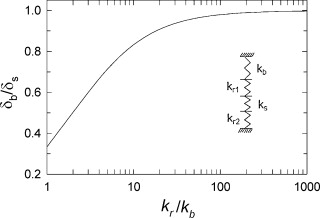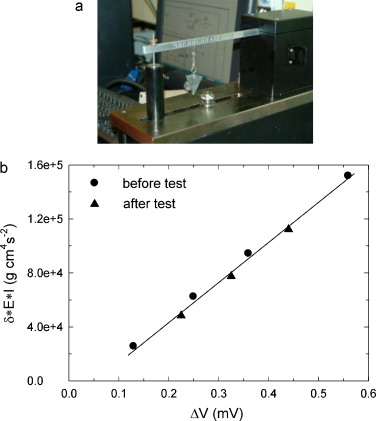# Analyses of a cantilever-beam based instrument for evaluating the development of polymerization stresses

## Objective

This investigation was to generate (1) guidelines for designing a tensometer that satisfies the necessary accuracy and sensitivity requirements for measuring polymerization stress (PS), and (2) a formula for calculating PS. Polymerization stress remains one of the most critical properties of polymeric dental materials, yet methods that can accurately quantify PS have been limited in part due to the complexity of polymerization, and in part due to the instrumentation itself.

## Method

In this study, we performed analytical and finite element analyses on a cantilever-beam based tensometer that is used to evaluate shrinkage stresses during the polymerization of dental restorative composites.

## Results

The PS generated by a commercial dental composite determined using our new tensometer agrees with the predicted trend when the beam length and/or specimen height is varied.

## Significance

This work demonstrates the importance of beam dimension and component relative rigidity to the accuracy of PS evaluation. An analytical solution is also derived for the vertical beam deflection, which can be used for any combination of bending and shearing to properly calculate the PS. In addition, an easy-to-conduct calibration procedure is provided that is desirable for periodic tensometer recalibration.

## Introduction

Human tooth structures (both their mineral and proteinaceous components) are adversely affected by caries, and the resulting cavities usually do not fully regenerate. Therefore, they require reconstruction utilizing adhesive and restorative and dental materials. Polymeric dental composites have been used widely in the restoration of tooth decay or cavity that occurs by primary caries or traumatic events. These materials, along with appropriate adhesive systems, are noted for their efficacy in restoring the function and appearance of tooth structure. However, failure of these dental restorations due to secondary caries is a major concern, and replacement of failed restorations creates extra pain, anxiety, and economic burdens for the patients . Dental restorations fail for a variety of reasons . For example, the stresses magnified (stress singularity) at or near the tooth/composite interface due to the mismatch of tooth and restorative mechanical properties are important contributors to failure. The polymerization shrinkage that occurs during the composite curing process has been implicated as a major stress source for the interfacial stress singularity . This shrinkage can lead to marginal microcracks and subsequent microleakage at or near the composite-tooth interface, permitting bacteria to pass beneath the restoration surface and ultimately resulting in the secondary caries.

Polymerization stresses (PS) of resin-based dental materials have been measured using a variety of methods. Those interested in methods traditionally used to quantify the PS of polymeric dental materials may refer to the existing literature . In general, the development of PS is measured through perturbing physically constrained specimens, and the resulting PS can be deduced through a corresponding governing equation for the response of constraints to the perturbation. Our interest here is not to propose a new method or to compare the relative merits of existing methods. Instead, the aim of this study is to assess and improve a widely used cantilever-beam based instrument in terms of the applicability of a formula for deducing PS from the constrained polymerization shrinkage. The necessity of this type of measurement is that the instrument be sufficiently sensitive to accurately detect shrinkage, manifested in beam deflection.

The aforementioned tensometer was designed and constructed by the American Dental Association Foundation (ADAF) located at the National Institute of Standards and Technology . Using this device, composite (or resin) specimens are mechanically attached to a cantilever beam via a quartz rod adhesively in contact with the specimen, and the specimen is also adhesively attached to a fixed lower rod. Upon polymerization, the composite shrinkage stress induces a deflection in a calibrated cantilever beam, and the beam deflection is measured using a linear variable differential transformer (LVDT). The PS is calculated through a beam formula according to the measured deflection . The original publication also noted that the level of tensometer compliance can be conveniently adjusted by varying the specimen position along the beam span to match that of a variety of clinically relevant restorative configurations. In a separate work, a similar tensometer was coupled to an IR soectrometer and used to determine the conversion dependent development of shrinkage and stress . In addition, the relationships between PS development and the physical/chemical evolution of network structure associated with dental polymers were examined .

In this study, the importance of relative rigidities between tensometer components and the testing materials on the instrument sensitivity is demonstrated. As a result, revised design criteria have been proposed for instrument fabrication to achieve more reliable measurements. Also, we have expanded the formula for extracting PS from the tensometer response through the elasticity theory when beam shearing is important. This formula has been validated by finite element analysis. A tensometer constructed according to the new design criteria was used to quantify PS by varying beam locations and heights. By comparing the analytical solution with experimental results, the new instrument gives the correct trend in PS development as a function of beam length. The same trend is not attainable from the original instrument . In addition, a very simple, straightforward, and portable calibration method is developed; this simple calibration method can avoid extra instrumental setups and unnecessary experimental procedures.

## Theory

The fundamental concept governing the operation of a cantilever-beam based tensometer is that PS is related to beam deflection . Fig. 1 a presents a photograph of the typical tensometer setup. The composite specimen in a cylindrical geometry is bonded to quartz rods via silane-coupling chemistry. The top surface of the upper rod (shrinkage-transmitting rod) is mechanically attached to the cantilever beam by means of a collet, while the lower rod is affixed to the base stand by a separate collet. As light transmits through the lower rod onto the specimen, the irradiation process initiates polymerization resulting in shrinkage, which exerts force causing the beam to deflect. LVDT is used to measure the beam deflection, δ ⋅ The force, F , is derived based on the configuration shown in Fig. 1 b through the following equation:

<SPAN role=presentation tabIndex=0 id=MathJax-Element-1-Frame class=MathJax style="POSITION: relative" data-mathml='F=6δEIa2(3ℓ−a)’>F=6δEIa2(3a)F=6δEIa2(3ℓ−a)
F = 6 δ E I a 2 ( 3 ℓ − a )

where ℓ and a are the distances between the clamped edge and the LVDT and sample location, respectively. E and <SPAN role=presentation tabIndex=0 id=MathJax-Element-2-Frame class=MathJax style="POSITION: relative" data-mathml='I(=wh3/12)’>I(=wh3/12)I(=wh3/12)
I ( = w h 3 / 12 )
are the Young’s modulus and the moment of inertia of the beam; h and w are the height and width of beam cross-section, respectively. The polymerization stress, σ , is simply the force divided by the cross-section area of specimen, A , as follows:

<SPAN role=presentation tabIndex=0 id=MathJax-Element-3-Frame class=MathJax style="POSITION: relative" data-mathml='σ=FA’>σ=FAσ=FA
σ = F AFig. 1

The formula shown in Eq. (1) is derived based on a linear elastic system and Euler–Bernoulli beam theory in which the vertical deflection of a beam is assumed to arise from bending while deflection due to shearing is negligible. Under these stated conditions, the beam length is much greater than the beam depth (large slenderness ratio, i.e., length/depth ratio (ℓ/ h )). It should be emphasized that the effective beam length (span) of the tensometer configuration shown in Fig. 1 b should be the length of “ a ”, where the sample is located and polymerization stress is exerted. Therefore, the aspect ratio of the beam in this study is defined as the ratio of effective beam length to beam height ( a / h ). Fig. 2 shows the displacements calculated using Eq. (1) and the analytical solution that includes bending and shearing at the LVDT location as a function of span/depth ratio for various values of a /ℓ. The analytical solution is derived from the elasticity theory that includes bending and shearing and will be discussed in more detail later. From Fig. 2 , it is apparent that the span/depth ratio should be 8 or greater for the contribution of beam shearing to the beam deflection (measured at the location of LVDT) to be negligible. Otherwise, the force deduced through Eq. (1) can be artificially low due to contributions from beam shearing. Also, results shown in Fig. 2 indicate the ratio of a /ℓ minimally affects the shearing contribution. In the experiment, the beam deflection at the sample position, δ s , should be less than half of the beam’s thickness (i.e., δ s / h < 0.5) to avoid in-plane stretching of the beam (diaphragm strain) and fulfill the requirements to satisfy linear elasticity. These requirements have been proven to be valid by our analyses using the finite element method in a previous study on the deformation of flexible membranes .Fig. 2

The tensometer system shown in Fig. 1 can be modeled as four springs in series (four components shown in the insert of Fig. 3 : beam, upper rod, specimen, and lower rod) during the beam deflection. The beam spring constant is k b (= 3 EI / a 3 ); the upper rod is k r 1 (= E r A /ℓ r 1 ), the specimen is k s (= E s A /ℓ s ), and the lower rod is k r 2 (= E r A /ℓ r 2 ), where E and ℓ are the modulus and length of each component. Subscripts r and s represent the rod and the sample, respectively; subscripts 1 and 2 refer to the upper and lower rods, respectively. Ideally, the measured beam deflection should reflect exclusively the specimen deformation due to shrinkage, and no rod deformation contributions. A commercial finite element code, SIMULIA , was employed to analyze the effect of relative rigidity of components on the beam deformation. The results shown in Fig. 3 indicate that the ratio of k r to k b should be relatively large (i.e., >100, assuming ℓ r 1 = ℓ r 2 ) so that the deformation of quartz rods becomes negligible. From the expression using the beam spring constant, it is clear that span/depth ratio ( a / h ) affects not only the applicability of Eq. (1) , but also the sensitivity of the beam to the presence of shrinkage.Fig. 3

## Instrument calibration

Besides fundamental requirements for instrument accuracy, a facile procedure to calibrate voltage to displacement is needed for periodic recalibration. A constant known weight (in this case a fishing sinker) is used as a load source ( P ) to characterize the force exerted on the beam ( Fig. 4 a ). For each hanging location along the beam from the clamped beam edge ( x ), an LVDT voltage reading (Δ V ) is recorded and a factorized beam displacement ( δ c = δ · E · I ) is calculated from the following equation:

<SPAN role=presentation tabIndex=0 id=MathJax-Element-4-Frame class=MathJax style="POSITION: relative" data-mathml='δc=Px2(3ℓ−x)6′>δc=Px2(3x)6δc=Px2(3ℓ−x)6
δ c = P x 2 ( 3 ℓ − x ) 6Fig. 4

As mentioned earlier, the ratio of x to h needs to be greater than eight (i.e., x / h ≥ 8) so that Eq. (3) is valid. Fig. 4 displays a typical δ c vs. Δ V calibration curve, which was generated by varying the sinker location along the beam; as expected a linear relationship is obtained. In a PS measurement, the force ( F , used in Eq. (2) ) corresponding to a sample position can be readily obtained through the following equation:

<SPAN role=presentation tabIndex=0 id=MathJax-Element-5-Frame class=MathJax style="POSITION: relative" data-mathml='F=6SΔVa2(3ℓ−a)’>F=6SΔVa2(3a)F=6SΔVa2(3ℓ−a)
F = 6 S Δ V a 2 ( 3 ℓ − a )
Only gold members can continue reading. Log In or Register to continue

Nov 28, 2017 | Posted by in Dental Materials | Comments Off on Analyses of a cantilever-beam based instrument for evaluating the development of polymerization stresses
Premium Wordpress Themes by UFO Themes